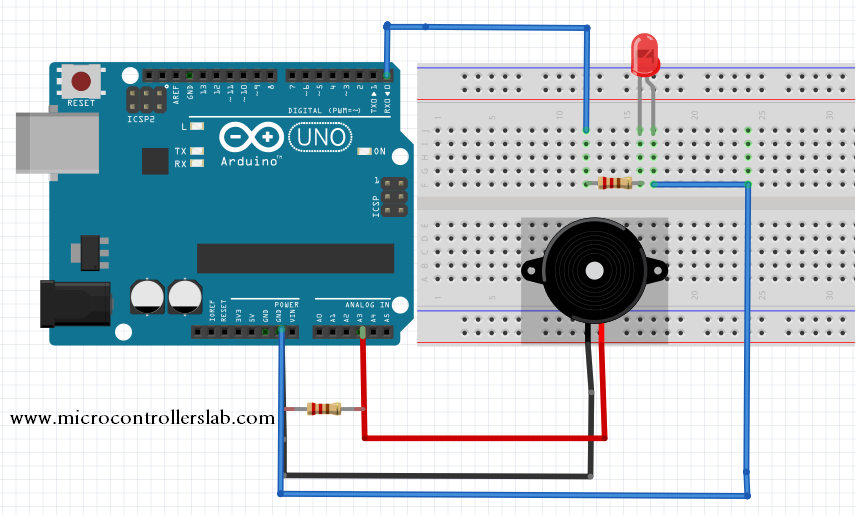# piezoelectric sensor interfacing with Arduino Uno R3

piezoelectric sensor interfacing with Arduino Uno R3, this is a student level / semester level project based on Arduino Uno R3 and piezoelectric sensor. In this project you will learn about how to interface piezoelectric sensor with Arduino Uno R3 ? What is piezoelectric sensor? What is output of piezoelectric sensor? How piezoelectric sensor works? How arduino respond to change in output of piezoelectric sensor?  Applications of piezoelectric sensor.I will also discuss both hardware connections of Arduino Uno R3 with piezoelectric sensor and programming to do all these tasks automatically.

#### What is piezoelectric sensor?

Piezoelectric sensor is basically a transducer which converts stress applied on it into some electrical energy. It is simply used the principle of energy conversion from one form to another.  As in case of piezoelectric sensor, it converts physical stress into electrical energy.  Stress can be a force , pressure , acceleration and touching potential. Because these all are kind of stresses and piezoelectric sensor converts such kind of physical forces into electrical form.

#### Application of piezoelectric sensor or transducer :

you will see enormous applications of piezoelectric transducer around you in daily usage things. Some of the major application of this sensor is given below :

• Motion detection
• Door knock sensor
• Acceleration measurement system
• force measurement system
• pressure measurement system
• Microphone used sound pressure to convert it into electrical form.
• lighters for cigarette
• testing of high voltage equipment’s
• And there are thousands of applications of piezo electric sensor.

#### How piezoelectric transducer or sensor works?

Whenever any stress is applied to piezoelectric sensors, a potential difference of same magnitude of force is produced across piezoelectric transducer. So it can be easily used to convert mechanical energy into electrical energy. Piezoelectric sensors produced analog output voltage. If you want to understand internal construction and working of this sensor. I recommend you to search it on Google. Because it is not possible for me to cover all the things in one topic. But if you know about basic working of piezoelectric transducer, it is enough to use in your project.

#### Piezoelectric sensor interfacing with Arduino Uno R3:

Piezoelectric sensors produce analog voltage at the output. So to interface this sensor with Arduino Uno R3, you should know how to measure analog voltage with Arduino Uno R3. If you don’t know how to measure analog voltage using Arduino, I suggest you to read following article first. So that you can learn Piezoelectric sensors interfacing with Arduino properly.

Diagram below shows the hardware connections of Piezoelectric sensor interfacing with Arduino. Piezoelectric sensors have two output pins one is positive potential and other is at negative potential ( means ground ). Positive potential pin is connected with pin 3 analog channel of Arduino and negative potential pin is connected to ground. A resistor of 2 mega ohm is connect between them  for protection purpose. A led is connected to pin zero to check working of sensor output.piezoelectric sensor interfacing with Arduino Uno R3

#### Code

The code given below is written using Arduino IDE
``` int sensoroutput = 4; // the analog pin connected to the sensor int ledoutput = 0; // pin connected to LED int THRESHOLD = 100; void setup() { pinMode(ledPin, OUTPUT);   // this function is used to declare led connected pin as output } void loop() { int value = analogRead(sensoroutput);  // function to read analog voltage from sensor if (value >= THRESHOLD)                    // function to check voltage level from sensor { digitalWrite(ledoutput, HIGH); delay(100); // to make the LED visible } else digitalWrite(ledoutput, LOW); } ```

### 7 thoughts on “piezoelectric sensor interfacing with Arduino Uno R3”

1. Is there a way to use one of these sensors to send a vailable signal to an electronic speed controller to controll how fast the motor goes?

2. Hi brother Malik,
My name is Yahya I am a student and we have to do a project in Biomedical Engineering. I have sent you an e-mail regarding power generation through walking using a piezoelectric device. I have also sent you a block diagram regarding it.

Would you please check it and could you please give suggestion. I would greatly appreciate it.

Thanks,

Yahya Ahmed

3. Hi I’m looking to produce a ultrasonic cleaning product and wonder if this code is applicable for both a ultrasonic sensor and transducer as you have talked about both above.